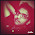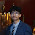Monday, January 12, 2015

Normal Shift and Lateral Shift due to Refraction of Light

Normal shift

When the object and the observer are in two different media,the image of the object appears like shifted from original position and it is called normal shift.

In this case we have to imagine the object in one medium and the observer in the other media. When we are seeing the object normally, image appears different from the position of the object. This is called normal shift.

When the object is in the denser medium and observer is in the rarer medium the first case is discussed.

When the light Ray moves from the object towards the observer, it has to move from wherever medium to denser medium. As it is moving from denser medium to rarer medium, it moves away from the normal and angle of refraction is more than that of the angle of incidence. Therefore for the observer the object appears nearer to the surface. This we can notice even in the real life also. If a coin is placed in a glass of water, for a human eye the coil appears nearer than where it is.

Similarly when the object is in the rarer medium and observer is in the denser medium it appears away from its original position and hence apparent height is more than that of the real height.

In both these cases we had calculated the shift of the image as shown.When the object is the denser medium,the image appears nearer to the surface and vice-versa.

Lateral shift on passing through a glass slab

Let us consider a light ray passing through a glass slab of certain thickness t. There is a change of the medium in this case twice. When the light Ray is entering from a into the glass slab it moves towards the normal. Again when it is moving away from the glass slab into the air it moves towards the normal.

This is happening as per the laws of refraction. According to the law when the light Ray is moving from rarer medium to denser medium it has to move towards the normal and vice versa.

After two reflections, it is quite possible that the emergent light Ray is parallel to the incident light Ray. The perpendicular distance between the two light rays within the glass slab is called lateral shift. We can determine the value of the lateral shift in terms of the thickness of the glass slab, angle of incidence and angle of refraction as shown below. Here we have used basic trigonometric definitions to get the required proof.

Problem and solution on normal shift

A mark at the bottom of a liquid appears to be rise by 0.1 m. The depth of the liquid is one meter. What is the refractive index of the liquid with respect to vacuum?

The object is in the denser medium and observer is in the rarer medium. As the light ray is passing from the denser medium rarer medium it moves towards the normal. Therefore for the observer the coin appears nearer to the surface by a given value. What is given in the problem means the apparent shift in the path with respect to the normal direction. Basing on definition and the derivation that we made in the previous post we can derive the equation and solve the problem as shown below.

The above diagram is also having one more problem.

A underwater swimmer is at a depth of 12 meter from the surface of water. The bird is at a height of 18 meter from the surface of water directly about his eyes. At what totally height does the swimmer locates the bird?

Here swimmer is the observer and the birdies the object. As the observer is in the rarer medium and the object is in the denser medium, for the observer object appears for than where it is. Using the concept of apparent shift we can solve the problem as shown above.

Problem one lateral shift

Related Posts

1.2.Well explained

3.More solved numericals on lateral shift please

4.More solved numericals on lateral shift

5.6.Nicely Explained...Thank You!

7.Nicely Explained...Thank You!

8.Nicely Explained...Thank You!
More solved numericals on lateral shift